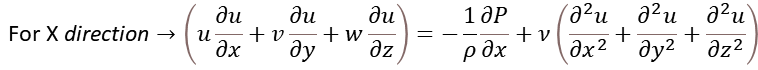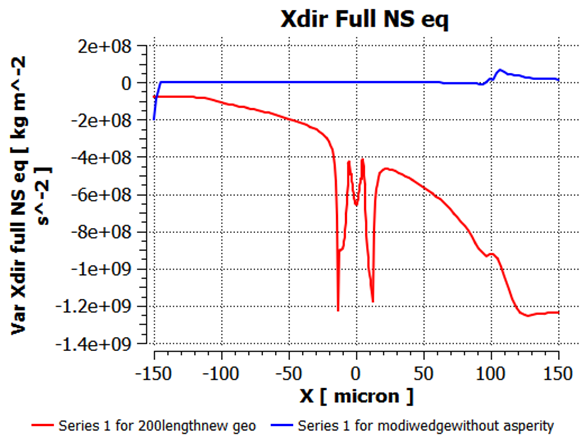## Fluids

Tagged:

•rupatel
Subscriber
I have solved a cavitation mixture model with laminar, translating the wall (flow direction) for 3d geometry. Its a flow between nonconformal contacting bodies. nI checked each term of Navier Stokes equation on streamline and equating addition of inertia to Pressure and viscous forces. I am getting errors near-wall boundary. However, if streamline is at the fluid domain the inertia forces are equal to generated pressure and viscous forces, but near solid wall its not. ncan someone tell me why is this difference, as my solution has converged fully, this means it should solve the Navier stokes equation completely and should not give me any residual as it not giving on the fluid domain, but its giving an error near the solid wall?.
•Karthik R
•rupatelwhen I see the Scaled residual(Continuity, x,y,z, velocity) in fluent, it converged. And I also plotted the convergence history of the Maximum pressure, which also started becoming constant after 500 iterations. On the basis of these plots generated by fluent, I consider my results have converged. Now, I export all solution data to CFD post-processing and trying to subtract inertia forces (L.H.S of the above equation) with RHS, which is the simplified form of Navier stokes equation according to my model, after applying the assumption. And checking results on the streamline. which is passing near the solid (red). I should get 0 similar to the blue line.
•rupatel
•DrAmine